# Geometrical transformations of images¶

## Cropping, resizing and rescaling images¶

Images being NumPy arrays (as described in the A crash course on NumPy for images section), cropping an image can be done with simple slicing operations. Below we crop a 100x100 square corresponding to the top-left corner of the astronaut image. Note that this operation is done for all color channels (the color dimension is the last, third dimension):

```>>> from skimage import data
>>> img = data.astronaut()
>>> top_left = img[:100, :100]
```

In order to change the shape of the image, `skimage.color` provides several functions described in Rescale, resize, and downscale .

```
from skimage import data, color
from skimage.transform import rescale, resize, downscale_local_mean

image = color.rgb2gray(data.astronaut())

image_rescaled = rescale(image, 0.25, anti_aliasing=False)
image_resized = resize(image, (image.shape // 4, image.shape // 4),
anti_aliasing=True)
image_downscaled = downscale_local_mean(image, (4, 3))

```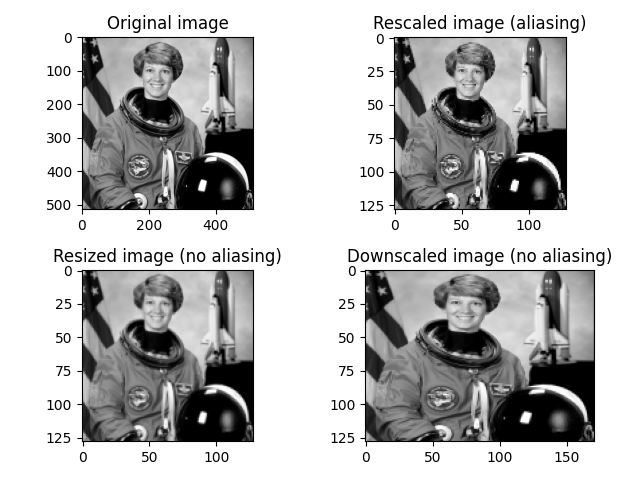## Projective transforms (homographies)¶

Homographies are transformations of a Euclidean space that preserve the alignment of points. Specific cases of homographies correspond to the conservation of more properties, such as parallelism (affine transformation), shape (similar transformation) or distances (Euclidean transformation). The different types of homographies available in scikit-image are presented in Types of homographies.

Projective transformations can either be created using the explicit parameters (e.g. scale, shear, rotation and translation):

```from skimage import data
from skimage import transform
from skimage import img_as_float

tform = transform.EuclideanTransform(
rotation=np.pi / 12.,
translation = (100, -20)
)
```

or the full transformation matrix:

```from skimage import data
from skimage import transform
from skimage import img_as_float

matrix = np.array([[np.cos(np.pi/12), -np.sin(np.pi/12), 100],
[np.sin(np.pi/12), np.cos(np.pi/12), -20],
[0, 0, 1]])
tform = transform.EuclideanTransform(matrix)
```

The transformation matrix of a transform is available as its `tform.params` attribute. Transformations can be composed by multiplying matrices with the `@` matrix multiplication operator.

Transformation matrices use Homogeneous coordinates, which are the extension of Cartesian coordinates used in Euclidean geometry to the more general projective geometry. In particular, points at infinity can be represented with finite coordinates.

Transformations can be applied to images using `skimage.transform.warp()`:

```img = img_as_float(data.chelsea())
tf_img = transform.warp(img, tform.inverse)
```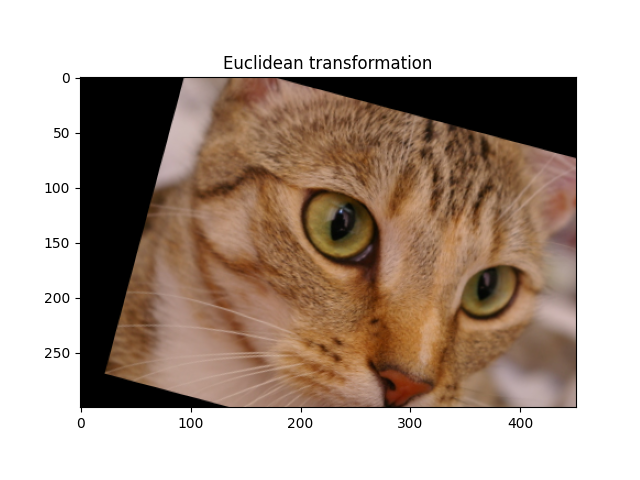The different transformations in `skimage.transform` have a `estimate` method in order to estimate the parameters of the transformation from two sets of points (the source and the destination), as explained in the Using geometric transformations tutorial:

```text = data.text()

src = np.array([[0, 0], [0, 50], [300, 50], [300, 0]])
dst = np.array([[155, 15], [65, 40], [260, 130], [360, 95]])

tform3 = transform.ProjectiveTransform()
tform3.estimate(src, dst)
warped = transform.warp(text, tform3, output_shape=(50, 300))
```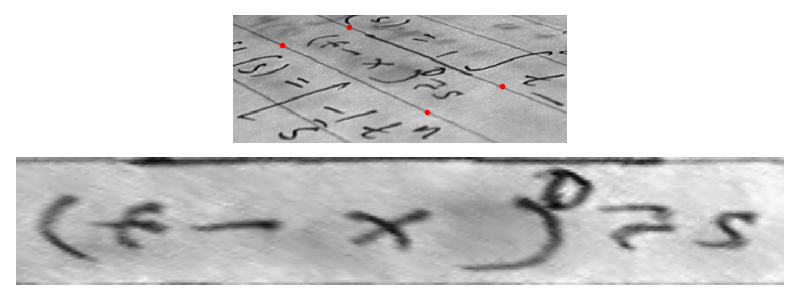The `estimate` method uses least-squares optimization to minimize the distance between source and optimization. Source and destination points can be determined manually, or using the different methods for feature detection available in `skimage.feature`, such as

and matching points using `skimage.feature.match_descriptors()` before estimating transformation parameters. However, spurious matches are often made, and it is advisable to use the RANSAC algorithm (instead of simple least-squares optimization) to improve the robustness to outliers, as explained in Robust matching using RANSAC.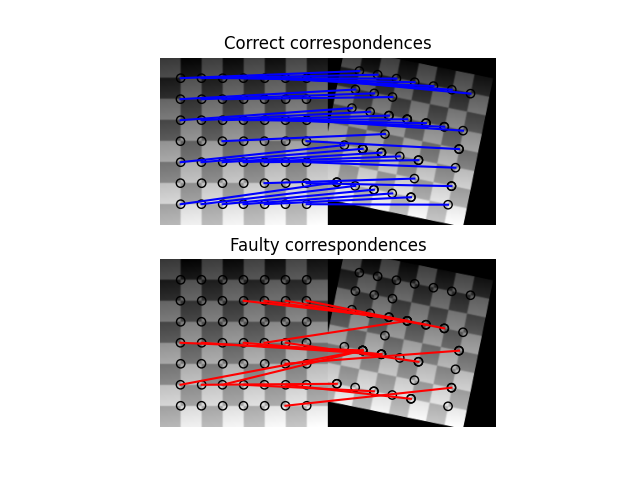Examples showing applications of transformation estimation are

The `estimate` method is point-based, that is, it uses only a set of points from the source and destination images. For estimating translations (shifts), it is also possible to use a full-field method using all pixels, based on Fourier-space cross-correlation. This method is implemented by `skimage.registration.register_translation()` and explained in the Image Registration tutorial.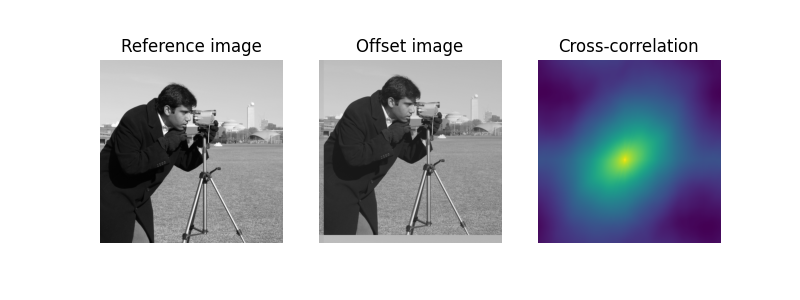The Using Polar and Log-Polar Transformations for Registration tutorial explains a variant of this full-field method for estimating a rotation, by using first a log-polar transformation.